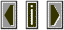Sound Pressure LevelSound pressure level is a measurement of the pressure of a sound in relation to a fixed reference point.  The reference for this measurement is the threshold of hearing which is  10**-12 watts/m**2  and is given a value of 0 dB.  Since the square of the sound pressure is proportional to the sound's intensity the equation for calculating sound pressure level is much the same as for sound intensity, only taking the square of the proportions.
SPL = 10 log(p/pref)**2 = 20 log (p/pref)